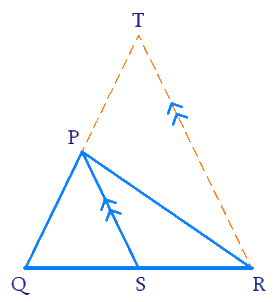# Ex. 6.6 Q1 Triangles Solution - NCERT Maths Class 10

## Question

In below Fig, $$PS$$ is the bisector of $$\angle {{QPR}}$$ of $$\Delta PQR$$. Prove that \begin{align}\frac{{QS}}{{SR}} = \frac{{PQ}}{{PR}}\end{align}Video Solution
Triangles
Ex 6.6 | Question 1

## Text Solution

Reasoning:

If a line is drawn parallel to one side of a triangle to intersect the other two sides in distinct points, the other two sides are divided in the same ratio.(BPT)

Steps:

Draw a line parallel to $$PS,$$ through $$R,$$ which intersect $$QP$$ produced at $$T$$

$PS\parallel RT$

In $$\Delta QPR$$

\begin{align}&\angle QPS = \angle SPR \\ &\text{(PS is bisector of }\angle QPR \; \dots (1) \end{align}

But,

\begin{align}& \angle PRT = \angle SPR \\ &\text{(Alternate interior angles)}\; \dots (2) \end{align}

\begin{align}& \angle QPS = \angle PTR \\ &\text{(Corresponding angles)}\; \dots (3) \end{align}

From $$\rm{(1), (2)}$$ and $$\rm{(3)}$$

\begin{align}\angle PTR &= \angle PRT\\ PR &= PT\;.......\rm (4)\end{align}

(Since in a triangle, sides opposite to the equal angles are equal)

In $$\Delta QSP,PS \parallel RT$$

\begin{align}\frac{{QS}}{{SR}}&= \frac{{QP}}{{PT}}\;\;\;\left[ {{\rm{BPT}}} \right]\\\frac{{QS}}{{SR}}&= \frac{{QP}}{{PR}}\;\;\;\left[ {{\rm{from (4)}}} \right]\end{align}

Learn from the best math teachers and top your exams

• Live one on one classroom and doubt clearing
• Practice worksheets in and after class for conceptual clarity
• Personalized curriculum to keep up with school